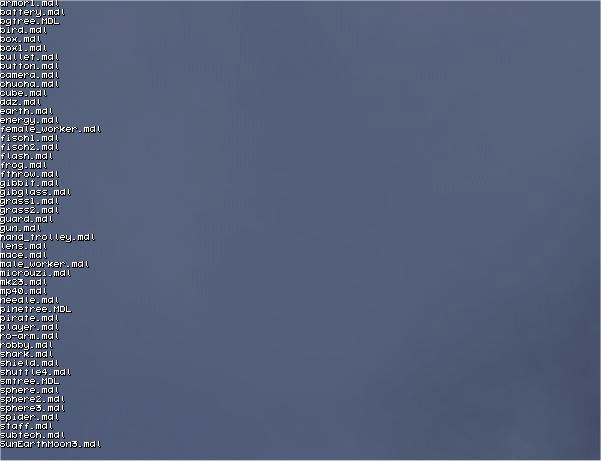New engine features
 txt_sort (text)   Sorts all strings of a text in ascending, alphabetical order (case insensitive).   text models_txt {        strings = 50; // display up to 50 models        flags = visible; }   starter check_models() // get the names for all the models in the current folder {        txt_for_dir(models_txt, "*.mdl"); // loads the model names        txt_sort (models_txt); // sort the names }draw_point3d (position, color, alpha, size)   Writes a point (a colored dot) at the given position in the level. Useful for special effects or for testing / debugging.   starter draw_points() {        while (1)        {                draw_point3d (vector (0, 0, 200), vector (255, 0, 0), 80, 7);                draw_point3d (vector (50, 0, 200), vector (0, 255, 0), 80, 7);                draw_point3d (vector (0, 50, 200), vector (0, 0, 255), 80, 7);                draw_point3d (vector (50, 50, 200), vector (255, 255, 255), 80, 7);                wait (1);                } }path_name   Gives the file name (including the path) of the last file that was opened by the engine. Can be used to find the path of an entity, image, or sound file that was opened by ent_create or file_load.   string model_str = ;   text path_txt {        pos_x = 30;        pos_y = 50;        string = path_name;        flags = visible; }   function create_model() {        ent_create (model_str, vector (random(1000), random(1000), random(1000)), null); }   on_c = create_model;